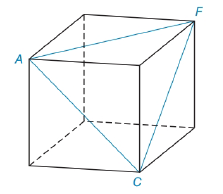Chapter 5.4, Problem 39EElementary Geometry For College St...

7th Edition
Alexander + 2 others
ISBN: 9781337614085

Solutions

Chapter
SectionElementary Geometry For College St...

7th Edition
Alexander + 2 others
ISBN: 9781337614085
Textbook Problem

A, C and F are three of the vertices of the cube shown in the accompanying figure. Given that each face of the cube is a square, what is the measure of angle ACF?To determine

To Find:

The angle ACF.

Explanation

Given A, C and F are three of the vertices of the cube shown in the accompanying figure. Given that each face of the cube is a square,

From then figure, observe that each side of triangle ACF is diagonal of one square. Since this is a cube, then the square on each face are congruent. Hence the diagonal of them are congruent. It follows that

AC¯AF¯x

Still sussing out bartleby?

Check out a sample textbook solution.

See a sample solution

The Solution to Your Study Problems

Bartleby provides explanations to thousands of textbook problems written by our experts, many with advanced degrees!

Get Started

Find a formula for the inverse of the function. 22. f(x)=4x12x+3

Single Variable Calculus: Early Transcendentals, Volume I

In Problems 27-30, write the equation of the tangent line to each curve at the given point. 29.

Mathematical Applications for the Management, Life, and Social Sciences

Evaluate the integral. 1x24x+1dx

Calculus (MindTap Course List)

Suppose that P(E)=.60, P(F)=.32, and P(EF)=.22. Are E and F independent?

Finite Mathematics for the Managerial, Life, and Social Sciences